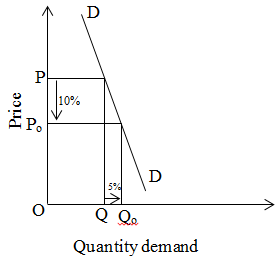# Relatively inelastic demand

If the percentage change in quality demand is percentage change in price is known as relatively inelastic demand. For example 5% change in quantity demand due to 10% change in price. In the case of normal good, demand is relatively inequality. We can explain by the given figure.On the above figure, when price is OP then quantity demand is OQ. When price decreases by 10% to Po then quantity demand is OQ1. It is increased by 5%.